Next: 6.4 Sharp Interface and Up: 6. Simulation of Thermal Previous: 6.2 Simulation Procedure

# 6.3 Meshing Aspects

The mesh generation for the (oxidation) simulation with FEDOS is an important topic, because the finite element formulation depends on the used elements. With the actual FEDOS version the discretization can be performed only with linear shape functions on tetrahedrons. This tetrahedral grid generation is performed with the in-house meshing tool LAYGRID from the Smart Analysis Programs package . The quality of the numerical solution of the PDE s by the finite element method increases with the number of nodes.

For a desired high accuracy of the simulation results a fine mesh with a high number of elements and nodes is requested . If the mesh is not fine enough, there is a risk that the Newton method does not converge for the discretized non-linear equation system because of too large approximation failure. On the other side a large number of elements and nodes has an unwanted effect: more computer resources are required, because it must be iterated over more elements which also must be assembled to the global equation system. In case of the oxidation model there are five variables on each node, so that an additional node results in five additional equations. A larger equation system needs more time and memory for its solving. Therefore, the goal for finite elements is always to obtain a high accuracy with the smallest possible number of elements.

For the oxidation simulation a static grid is used which has the advantage that grid manipulation procedures are not needed. A grid modification like refining and coarsening in each time step normally needs complex algorithms with a long computation time and has the risk of element degeneration . So the best way to reach the above goal with a static grid is to make an initial mesh with appropriate local resolution. In critical or intersting regions of the investigated structure, or where the oxidation process really occurs, a finer mesh should be applied than in the rest of the structure.

The previously discussed meshing strategy is applied to discretize an initial structure as shown in Fig. 6.3. This demonstrative example is a silicon block with (1.2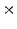0.3)m floor space and a height of 0.4m. Two thirds of the length are covered with a 0.15m thick silicon nitride mask which prevents the oxidant diffusion on the subjacent silicon block. In principle this chosen structure is two-dimensional, but it is very suitable for the plausible illustration how the sharp interface interpretation (see Section 6.4) and the stress calculation strategy (see Section 7.2) works. The first interesting information regarding meshing is that the oxidation process only starts at the upper uncovered silicon surface. The next important aspect is that the most critical region on this structure is along the edge of the Si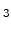N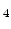-mask. This area is of interest, because the stiffness of the SiN-mask prevents the desired volume expansion of the newly formed oxide, which leads to the well known bird's beak effect.

Therefore, the finest mesh in the structure was constructed around the mask edge. The distance of the nodes in the x-direction is 10nm. For nodes which are located away located from the edge their distance is successively increased until 50nm at the end of the active silicon region (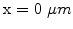). At the end of the SiN-mask (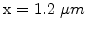) the node distance in x-direction is even 100nm. Furthermore, on the upper half of the silicon block where the oxidation process is expected the layer thickness is 10nm, in the lower half it is doubled (20nm). Unfortunately LAYGRID is limited to produce only layers with constant thickness over the whole x-y-plane. Therefore, the layer thickness in the less interesting regions somewhere under the mask must be the same as in the active area. All in all, the mesh shown in Fig. 6.3 has 12218 nodes and 56670 (tetrahedral) elements.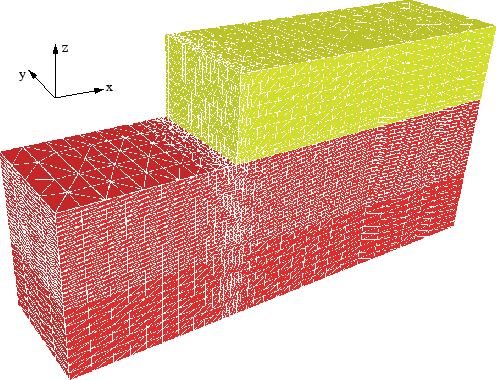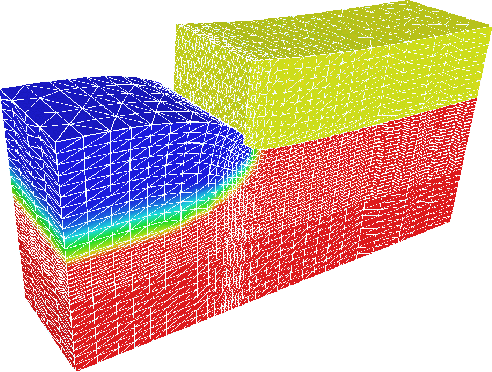The results of the simulated oxidation process, which are the-distribution and the displacements in the materials, are displayed in Fig. 6.4. Here, blue is pure SiO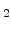(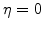), red is the pure silicon substrate (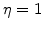), and at the Si/SiO-interface one can see the reaction layer with a finite width (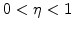) as explained in Section 3.1. Furthermore, this figure depicts the node displacements and the grid deformation caused by the considerable volume increase of the newly formed SiO.Next: 6.4 Sharp Interface and Up: 6. Simulation of Thermal Previous: 6.2 Simulation Procedure

Ch. Hollauer: Modeling of Thermal Oxidation and Stress Effects